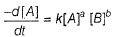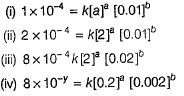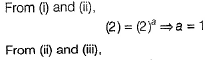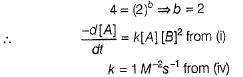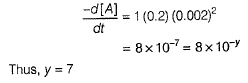Courses

# Test: Determination of Rate of Reaction

## 24 Questions MCQ Test Chemistry for JEE | Test: Determination of Rate of Reaction

Description
This mock test of Test: Determination of Rate of Reaction for JEE helps you for every JEE entrance exam. This contains 24 Multiple Choice Questions for JEE Test: Determination of Rate of Reaction (mcq) to study with solutions a complete question bank. The solved questions answers in this Test: Determination of Rate of Reaction quiz give you a good mix of easy questions and tough questions. JEE students definitely take this Test: Determination of Rate of Reaction exercise for a better result in the exam. You can find other Test: Determination of Rate of Reaction extra questions, long questions & short questions for JEE on EduRev as well by searching above.
QUESTION: 1

### Direction (Q. Nos. 1-13) This section contains 13 multiple choice questions. Each question has four choices (a), (b), (c) and (d), out of which ONLY ONE is correct. For a given reaction, A → Product, rate = 1 x 10-4 Ms-1        at [A] = 0.01 M rate = 1.41 x 10-4   Ms-1  at [A] = 0.02 M Hence, rate law is

Solution:

Let rate law be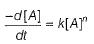1 x 10-4 = K(0.01)n

1.41 x 10-4 = K(0.02)n

∴ 1.41 = (2)n

(2)1/2 = (2)n   (Since, √2 = 1.41)

∴ n= 1/2

Thus rate law is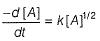QUESTION: 2

### The rate law for a reaction between the substances A and 8 is given by rate = k[A]n [B]m If concentration of A is doubled and that of B is halved, the new rate as compared to the earlier rate would be

Solution: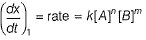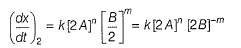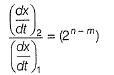QUESTION: 3

### The rate equation for the reaction, 2A + B → C is found to be, rate = k[A] [B] Q. The correct statement in relation to this reaction is that the

Solution:

Rate = k (A)[B]

The given reaction is first order in A and first order is B.
Thus, total order = 2

(a) Unit of k = cone 1 - n time -1 = conc-1 time-1 Thus, (a) is false.
(b)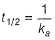of second-order reaction, thus (b) is false.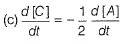Thus, (c) is correct.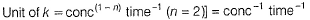Thus, value of k is dependent on the concentration of A and B. Thus, (d) is false.

QUESTION: 4

For the reaction,

2 A+B → Product

The half-life period was independent of concentration of B. On doubling the concentration A, rate increases two times. Thus, unit of rate constant for this reaction is

Solution: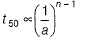t50 is independent of concentration of B then w.r.t. B, (n - 1) = 0. Thus, n = 1
Thus, order w.r.t. B = 1
Also, if concentration is made m-times, rate becomes, mn-times.

Hence, w.r.t.A

m = 2, m= 2

∴ 2n = 2 = 21

Thus  n = 1
Thus, order w.r.t. A = 1
Thus, total order = 2 = n
Unit of n th order reaction = conc(1-n) time-1
conc in mol L-1 and time in seconds.

Then,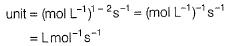QUESTION: 5

Which of the following statements appears to the first order reaction?

2N2O5(CCl4) → 4NO2(CCl4) + O2(g)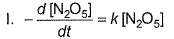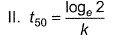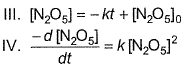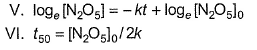Solution:

(a)

I, II, V - represent first order equation

III, VI - zeroth order equation

IV - second order equation

QUESTION: 6

For a reaction, time of 75% reaction is thrice of time of 50% reaction. Thus, order of the reaction is

Solution:

For nth order reaction (n > 1)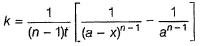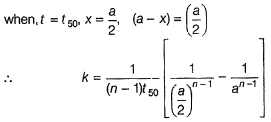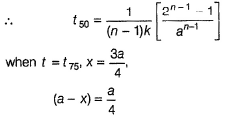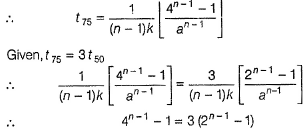This equation is true, if n = 2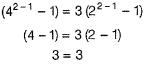QUESTION: 7

For nth order reaction,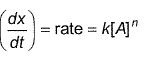Graphs between log (rate) and log[A0] are of the type ([A0] is the initial concentration)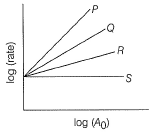Lines P, Q, R and S are for the order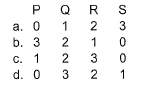Solution: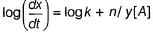n = order of the reaction =slope of the line
Larger the value of the slope of the line, larger the order.
Thus, P = 3, Q = 2, R = 1, S = 0.

QUESTION: 8

nA → Product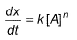For the reaction, rate constant and rate of the reaction are equal, then on doubling the concentration of A, rate becomes,

Solution: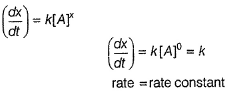Thus, order = zero Rate is independent of concentration of A.
Thus, rate remains constant.

QUESTION: 9

For the simple reaction,

A → B

When [A] was changed from 0.502 mol dm-3 to 1.004 mol dm-3 half-life dropped from 52 s to 26 s at 300 K. Thus, order of the reaction is

Solution:

For nth order reaction,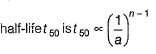(T50 ) is t1 ,at  [A] = aand is t2 at [A] = a2

∴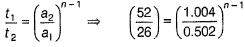(2) = (2)n-1

∴ n - 1 = 1, n = 2

QUESTION: 10

For the following reaction,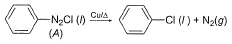Variation of T50 with [A] is shown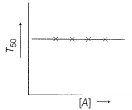Q.

After 10 min volume of N2 (g) is 10 L and after complete reaction, volume of N2 (g) is 50 L. Thus, T50 is

Solution:

T50 is independent of concentration of [A], Thus, it follows first order kinetics.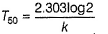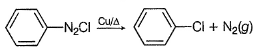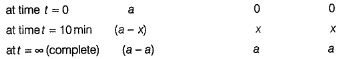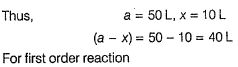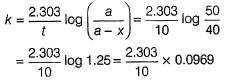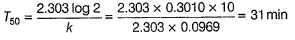QUESTION: 11

Kinetics of the following reaction,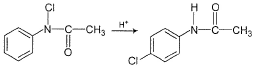can be studied by

Solution:

Cl-atom attached to N-atom, is an oxidising agent and can oxidise Kl to l2.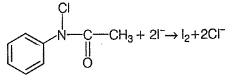l2 can be titrated using hypo in iodometric titration.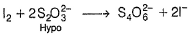Cl-atom attached to benzene nucleus is not an oxidising agent.

QUESTION: 12

Graph between [B] and time t of the reaction of the type I for the reaction, A → B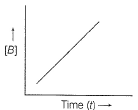Hence, graph between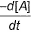and time t will be of the type

Solution:

A → B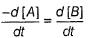[B] increases uniformly with time t. Order is zero. Thus, at every point,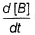= constant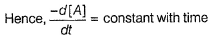QUESTION: 13

If in the fermentation of sugar in an enzymatic solution that is 0.12 M, the concentration of sugar is reduced to 0.06 M in 10 h and to 0.03 M in 20 h. Thus, order of the reaction is

Solution: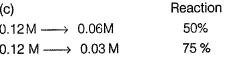We find that,    t75 = 2 x t50

Thus, given reaction is of first-order.

QUESTION: 14

Direction (Q. No. 14) Choices for the correct combination of elements from Column I and Column II are given as options (a), (b), (c) and (d), out of which one is correct.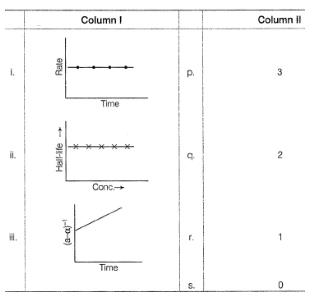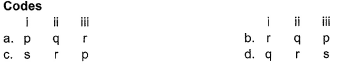Solution:

(i) Rate remains constant

(dx/dt) = k(A)n = [A]0 = k

Thus   order = 0

Thus,(i)→(s)
(ii) Half-life period is independent of concentration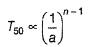∴  (n-1) = 0

∴  n = 1

Thus, (ii) →(r)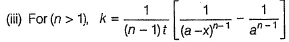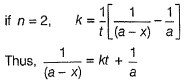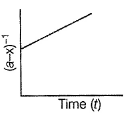Thus, graph is for second-order reactions

Thus, (iii) → (q)

QUESTION: 15

Direction (Q. Nos. 15-20) This section contains 3 paragraphs, each describing theory, experiments, data, etc. Six questions related to the paragraphs have been given. Each question has only one correct answer among the four given options (a), (b), (c) and (d).

Passage I

Peroxy acetyl nitrite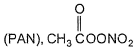is an air pollutant, as it decomposes into radicals :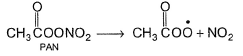A sample of polluted air is analysed for its PAN content which is reported as molecules per litre of air at 298 K.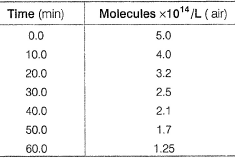Q.

Determine the order of the PAN decomposition

Solution:

0 min 5.0 x 1014  molecules L-1
30 min 2.5 x 1014 molecules L-1
60 min 1.25  x 1014 molecules L-1
At 30 min, 50% reaction takes place.
At 60 min, 75% reaction takes place.
Thus,   T75 = 2 x T50
it is valid for first order reaction. Thus, order = 1

QUESTION: 16

Passage I

Peroxy acetyl nitriteis an air pollutant, as it decomposes into radicals :A sample of polluted air is analysed for its PAN content which is reported as molecules per litre of air at 298 K.Q.

Rate constant of the reaction is

Solution:

Time : [PAN]

0 min 5.0 x 1014 molecules L-1

30 min 2.5  x 1014 molecules L-1

60 min  2.5 x 1014 molecules L-1
At 30 min, 50% reaction takes place.
At 60 min, 75% reaction takes place.

Thus,   T75 = 2 x t50

It is valid for first order reaction. Thus, order = 1

For first order reactions, T50 = 0.693/k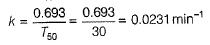QUESTION: 17

Passage II

H The reaction between nitric oxide (NO) and hydrogen (H2)has been investigated by measuring the initial rate of decrease of pressure in known mixture of gases. The following results were obtained at 1000 K.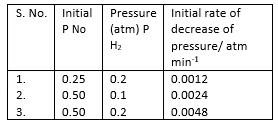Q.

Overall order of the reaction is

Solution:

-dp/dt = k(PNO)a (PH2)b

0.0012 = k(0.25)a(0.2)b

0.0024 = k(0.50)a(0.1)b

0.0048 = k(0.50)a(0.2)b

From Eqs. (i) and (iii), we get

4 = (2)a

∴   (2)2 = (2)a

∴   a = 2

Thus w.r.t. No, order = 2

From Eqs.)ii) and (iii), we get

2 = (2)b

(2)1 = (2)b

∴   b = 1
Thus, w.r.t. H2, order = 1

Total order = 3

From Eq. (i),

0.0012 =  k(0.25)2(0.2)= k (0.25)2(0.2)

∴  k = 0.0012/(0.25)2(0.02) = 0.096 atm-2 min-1

QUESTION: 18

Passage II

H The reaction between nitric oxide (NO) and hydrogen (H2)has been investigated by measuring the initial rate of decrease of pressure in known mixture of gases. The following results were obtained at 1000 K.Q.

Rate constant of the overall reaction is

Solution: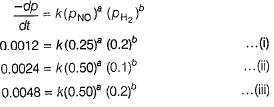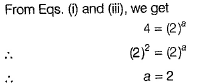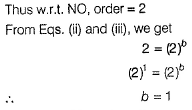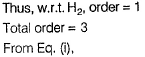0.0012 = k(0.25)2(0.2)1 = k(0.25)2(0.2)

∴   k = 0.0012/(0.25)(0.2) = 0.096 atm-2 min-1

QUESTION: 19

Passage III

A reaction between substances A and B is represented as:

A+B → C

Observations on the rate of this reaction are obtained as :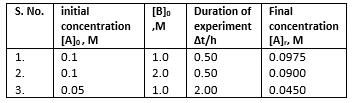Q.
Order of the reaction w.r.t. A and B respectively are

Solution: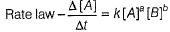where, a == order w.r.t. A, 6 = order w.r.t. B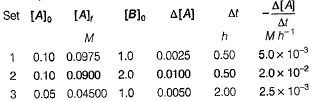(i) 5.0 x 10-3 = k[0.1]a[1.0]b

(ii) 2.0 x 1-2 = k [0.1]a[2.0]b

(iii) 2.5 x 10-3 = k [0.05]a[1.0]b

From Eqs. (i) and (ii), we get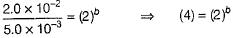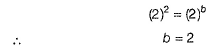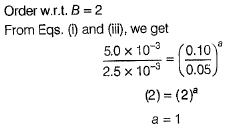Order w.r.t.A = 1

From Eq. (ii), 5.0x10-3 = K(0.1)(1.0)2

∴  k = 5.0 x 10-2M-2h-1

QUESTION: 20

Passage III

A reaction between substances A and B is represented as:

A+B → C

Observations on the rate of this reaction are obtained as :Q.

Rate constant of the overall reaction is

Solution:where, a == order w.r.t. A, 6 = order w.r.t. B(i) 5.0 x 10-3 = K [0.1]a [1.0]b

(ii) 2.0 x 10-2 = k [ 0.1]a[2.0]b

(iii) 2.5 x 10-3 = k [0.05]a[1.0]b

From Eqs. (i) and (ii), we get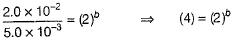(2)2 = (2)b

∴ b = 2

Order w.r.t.B = 2

From Eqs. (i) and (iii), we get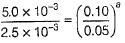(2) = (2)a

a = 1

Order w.r.t.A

From Eq. (ii),

5.0 x 10-3 = k (0.1) (1.0)2

∴ k = 5.0 x 10-2m-2h-1

*Answer can only contain numeric values
QUESTION: 21

Direction (Q. Nos. 21-24) This section contains 4 questions. When worked out will result in one integer from 0 to 9 (both inclusive).

Graph between logT50 (T50 is half-life period) and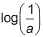(a = initial concentration) for n th order reaction is of the type.

What is the value of n?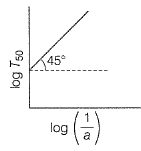Solution:

For nth order reactions,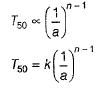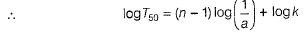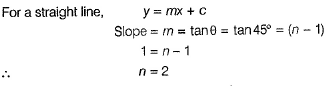*Answer can only contain numeric values
QUESTION: 22

The acid catalysed hydrolysis of an organic compound A at 30°C has a time for half change of 100 min when carried out in a buffer solution at pH 5 and 10 min when carried out at pH 4. Half-life in both cases are independent of initial concentration of A If rate law is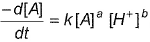, then what is the value of (a + b)?

Solution:

Half-life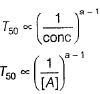T50 is independent of [A], then

(a-1) = 0

a = 1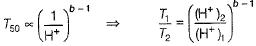T1 = 100 min, [H+]= 10-ph = 10-5M

T2 = 100 min, [H+]2 = 10-ph = 10-4M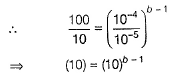b - 1 = 1

b = 2

∴ a + b = 3

*Answer can only contain numeric values
QUESTION: 23

For a reaction, A → B, half-life time for a reaction at [A] = 0.1M is 200 s and at [A] = 0.4M it is 50 s. Thus, order of the reaction i s ......

Solution: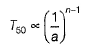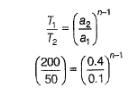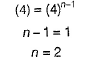*Answer can only contain numeric values
QUESTION: 24

For the reaction,

A + B → Product

Following four experiments were carried out: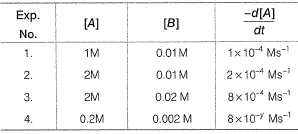Derive the value of y.

Solution: